# 骰子作画的算法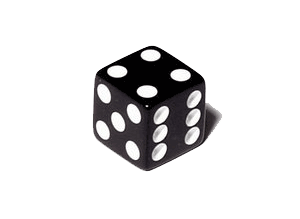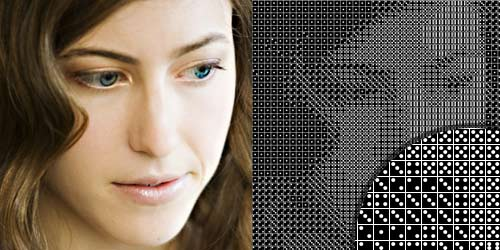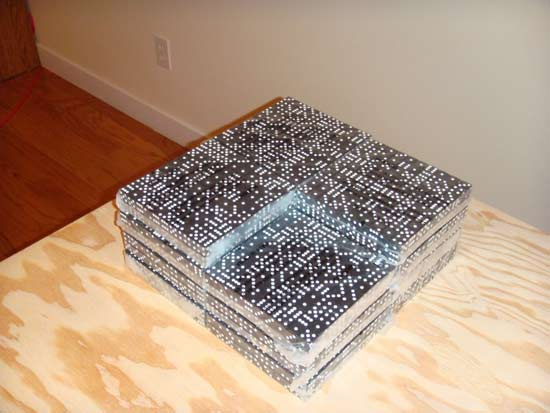for (int i=0; i < (pic_width/16); ++i) {

for (int j=0; j < (pic_height/16); ++j) {

patch = cropped_img.get(i*16, j*16, 16, 16);

}

}

for (int k=0; k < patch.pixels.length; ++k) {

x[k] = rgb2gray(patch.pixels[k]);

}

int rgb2gray(int argb) {

int _alpha = (argb >> 24) & 0xFF;

int _red = (argb >> 16) & 0xFF;

int _green = (argb >> 8 ) & 0xFF;

int _blue = (argb) & 0xFF;

return int(0.3*_red + 0.59*_green + 0.11*_blue);

}

int dice_num = six_step_gray(mean(x));

int mean(int[] x) {

float m = 0;

for (int i=0; i < x.length; ++i) {

m += x[i];

}

m = m/x.length;

return int(m);

}

int six_step_gray(int x) {

if (0 <= x && x <= 41) return 1;

if (41 < x && x <= 83) return 2;

if (83 < x && x <= 124) return 3;

if (124 < x && x <= 165) return 4;

if (165 < x && x <= 206) return 5;

if (206 < x && x <= 247) return 6;

else return 6;
}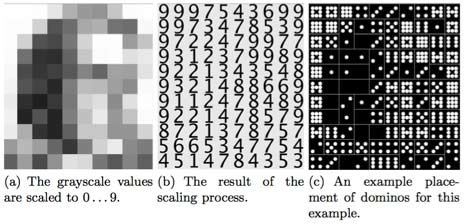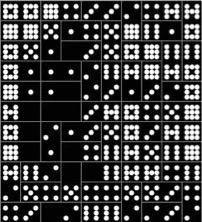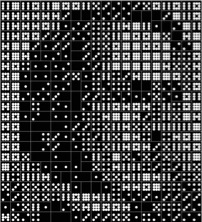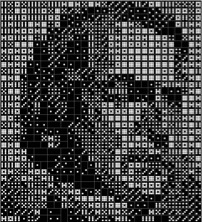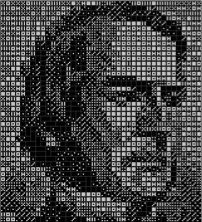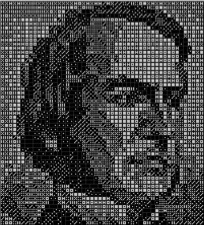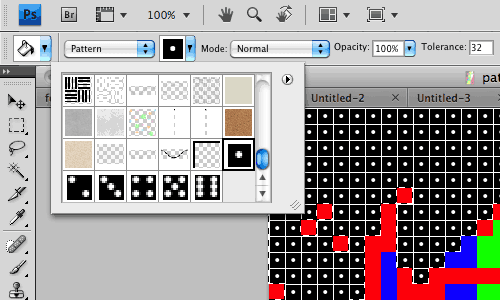（完）

## 留言（23条）

if (x < 206 && x <= 247) return 6;

`引用降落在心灵汤的鸡的发言：`

if (x < 206 && x <= 247) return 6;

PImage img;
PImage cropped_img;
PImage patch;

`引用up的发言：`

`引用ypchen的发言：`

`引用Brooklyn的发言：`

`引用牧马人的发言：`

«-必填

«-必填，不公开

«-我信任你，不会填写广告链接